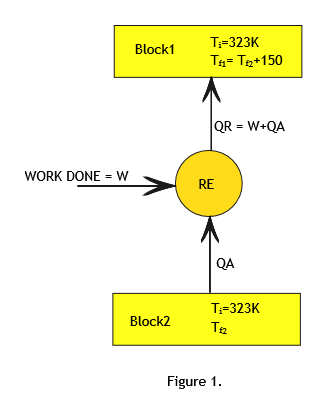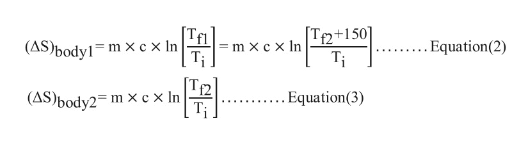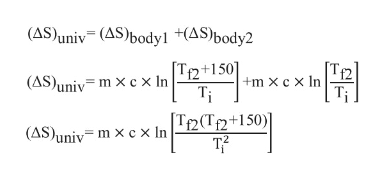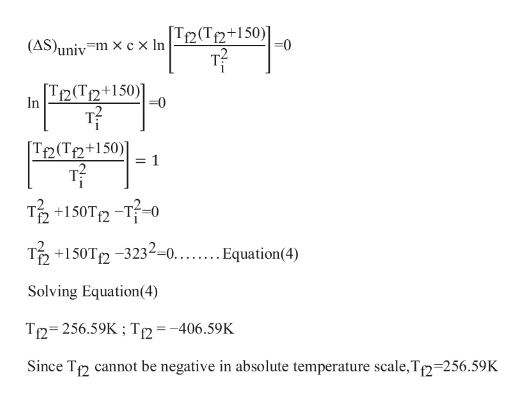Sample bartleby Q&A Solution
You ask questions, our tutors answer
Browse
Question

Two identical metal blocks of mass 20 kg and specific heat 0.4 kJ/kg are at an initial temperature of 50 degree Celsius .A reversible refrigerator receives heat from one block and rejects heat to the other. Find the work required by the refrigerator to cause a temperature difference of 150 degree Celsius between the two blocks.

## Expert Answer

Let us call the two blocks as block1 and block2. (Refer to Figure1)

Mass of each block (m)= 20kg.

Specific heat of each block (c)=0.4 kJ/kg.

Initial temperature of both the blocks is same (Ti)= 500C=323K.

Final Temperature of block1=Tf1.

Final Temperature of block2=Tf2.

Since heat is absorbed from block2 and  rejected to block1 ,Tf1 > Tf2  and it is given that Tf1 - Tf2 = 150.fullscreen

Since the refrigerator is reversible refrigerator all the processes involved are reversible processes.
According to Principle of entropy, for reversible processes change in entropy of the Universe is zero.fullscreen

Calculate change in entropy of body1 and body2 as follows:fullscreen

Substitute Equations (2) and (3) in Equation (1)fullscreen

Since change in entropy of the universe is zero for reversible processes, we havefullscreen

Calculate Heat Absorbed(QA) and Heat Rejected(QR) by the refrigerator as follows:

QA=mc (T- Tf2) = (20) (0.4) (323-256.59) = 531.28kJ

QR=mc (Tf1 - Ti) = (20) (0.4) (356.59-256.59) = 800kJ

Work done(WD)  on the refrigerator is calculated as follows:

Work Done(WD) = (QR) - (QA)

WD= 800 – 531.28 = 268.72kJ.

Refrigerator consumes 268.72kJ of work to cause a temperature difference of 150 Degree Celsius between the two blocks.